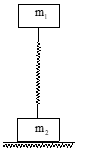Work done by Diff type of forces
Question

# Two plates whose masses are m1 and m2  respectively are connected by a massless spring as shown in figure.  What minimum force should be applied to the upper plate for it to lift the lower one after the force is removed.Moderate
Solution

## The lower plate will rise if spring force on it greater than its weight  ${\mathrm{Kx}}_{2}>{\mathrm{m}}_{2}\mathrm{g}$ suppose it is compressed by ${\mathrm{x}}_{1};\frac{1}{2}{\mathrm{Kx}}_{1}^{2}=\frac{1}{2}{\mathrm{Kx}}_{2}^{2}+{\mathrm{m}}_{1}\mathrm{g}\left({\mathrm{x}}_{1}+{\mathrm{x}}_{2}\right)$

Get Instant Solutions